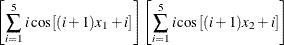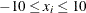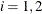### A Simple Example

The example that follows illustrates the application of genetic algorithms to function optimization over a real-valued domain. It finds the minimum of the Shubert function:wherefor.

proc ga seed = 12 maxiter = 30;
/* the objective function to be optimized */
function shubert(selected[*]);
array x /nosym;
x1 = x;
x2 = x;
sum1 = 0;
do i = 1 to 5;
sum1 = sum1 + i * cos((i+1)* x1 + i);
end;
sum2 = 0;
do i = 1 to 5;
sum2 = sum2 + i * cos((i+1) * x2 + i);
end;
result = sum1 * sum2;
return(result);
endsub;
/* Set the problem encoding */
call SetEncoding('R2');
/* Set upper and lower bounds on the solution components */
array LowerBound /nosym (-10 -10);
array UpperBound /nosym (10 10);
call SetBounds(LowerBound, UpperBound);
/* Set the objective function */
call SetObjFunc('shubert',0);
/* Set the crossover parameters */
call SetCrossProb(0.65);
call SetCross('Heuristic');
/* Set the mutation parameters */
call SetMutProb(0.15);
array del /nosym (0.2 0.2);
call SetMut('Delta','nchange', 1, 'delta',del);
/* Set the selection criteria */
call SetSel('tournament','size', 2);
call SetElite(2);
/* Initialize the first generation, with 120 random solutions  */
call Initialize('DEFAULT',120);
/* Now execute the Genetic Algorithm */
run;
quit;


At the beginning of the program, the PROC GA statement sets the initial random number seed and sets the maximum number of iterations to 30.

A routine to compute the objective function (function shubert) is then defined. This function is called by the GA procedure once for each member of the solution population at each iteration. Note that the GA procedure passes the array selected as the first parameter of the function, and the function uses that array to obtain the selected solution elements with a ReadMember call, which places the solution in the array x. The second parameter of the ReadMember call is 1, specifying that segment 1 of the solution be returned, which in this case is the only segment. The programming statements that follow compute the value of the objective function and return it to the GA procedure.

After the function definition, the 'R2' passed to the SetEncoding call specifies that solutions are single-segment, with that segment containing two elements that are real-valued. Next, a lower bound of –10 and an upper bound of 10 are set for each solution element with the SetBounds call. The SetObjFunc call specifies the previously defined Shubert function as the objective function for the optimization; the second parameter value of 0 indicates that a minimum is desired. The SetCrossProb call sets the crossover probability to 0.65, which means that, on average, 65% of the solutions passing the selection phase will undergo the crossover operation. The crossover operator is set to the heuristic operator by the SetCross call. Similarly, the mutation probability is set to 0.15 with the SetMutProb call, and the delta operator is set as the mutation operator with the SetMut call. The selection criteria are then set: a conventional tournament of size 2 is specified with SetSel call, and an elite value of 2 is specified with the SetElite call. The elite value implies that the best two solutions of each generation are always carried over to the next generation unchanged by mutation or crossover. The last step before beginning the optimization is the Initialize call. This call sets the population size at 120, and specifies the default initialization strategy for the first population. For real encoding, this means that an initial population randomly distributed between the upper and lower bounds specified in the SetBounds call is generated. Finally, when the RUN statement is encountered, the GA procedure begins the optimization process. It iterates through 30 generations, as set by the MAXITER= option.

The Shubert function has 760 local minima, 18 of which are global minima, with a minimum of –186.73. If you experiment with different random seeds with the SEED= option, PROC GA generally converges to a different global minimum each time. Figure 3.1 shows the output for the chosen seed.

Figure 3.1: Shubert Function Example Output

PROC GA Optimum Values

Objective
-186.7309031

Solution
Element Value
1 -7.708309818
2 -0.800371886